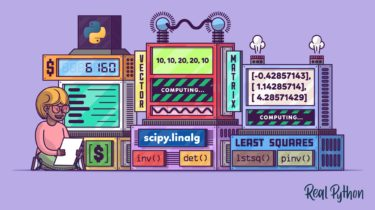# Linear Algebra in Python: Matrix Inverses and Least SquaresLinear algebra is an important topic across a variety of subjects. It allows you to solve problems related to vectors, matrices, and linear equations. In Python, most of the routines related to this subject are implemented in `scipy.linalg`, which offers very fast linear algebra capabilities.

In particular, linear models play an important role in a variety of real-world problems, and `scipy.linalg` provides tools to compute them in an efficient way.

In this tutorial, you’ll learn how to:

• Study linear systems using determinants and solve problems using matrix inverses
• Interpolate polynomials to fit a set of points using linear systems
• Use Python to solve linear regression problems
• Use linear regression to predict prices based on historical data

This is